# Discussion by Recall Network

Network of members that are discussed with by recall

The Discussion by recall network shows the members and advisors of the student government that are most often discussed with informally by recall.

## Examples

### Basic Examples

Retrieve the graph:

 In:=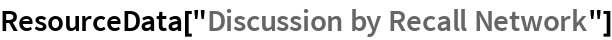Out=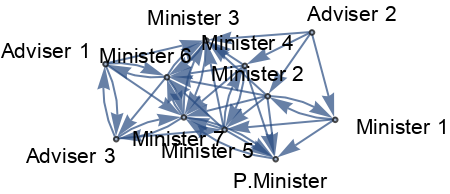Summary properties:

 In:=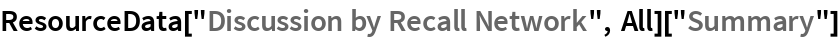Out=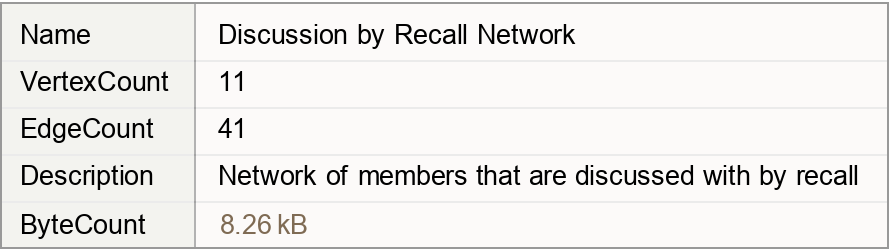### Basic Applications

Show the discussions between members:

 In:=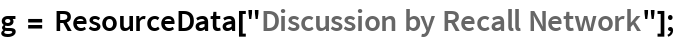In:=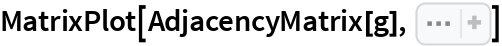Out=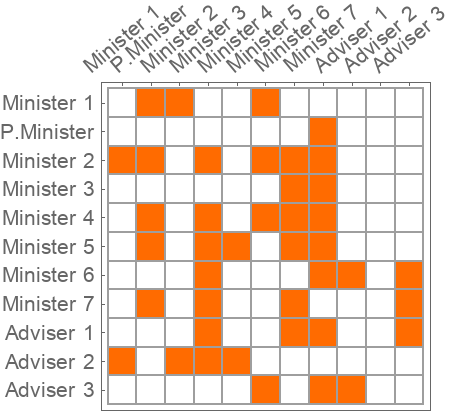Wolfram Research, "Discussion by Recall Network" from the Wolfram Data Repository (2019)